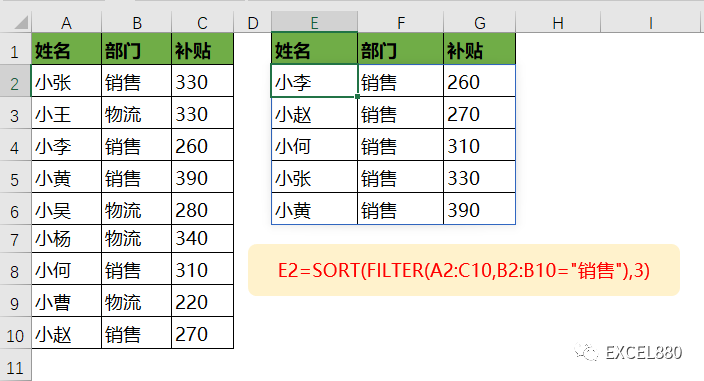# 放弃万金油，FILTER才是最强的查询筛选函数！

246 次浏览

FILTER函数是Excel 2021版本中的新函数之一，作用是筛选符合条件的单元格。

FILTER(要筛选啥数据？，按啥条件筛选？，[没有符合条件的数据时返回啥？])

=FILTER(A2:A10,B2:B10=E2)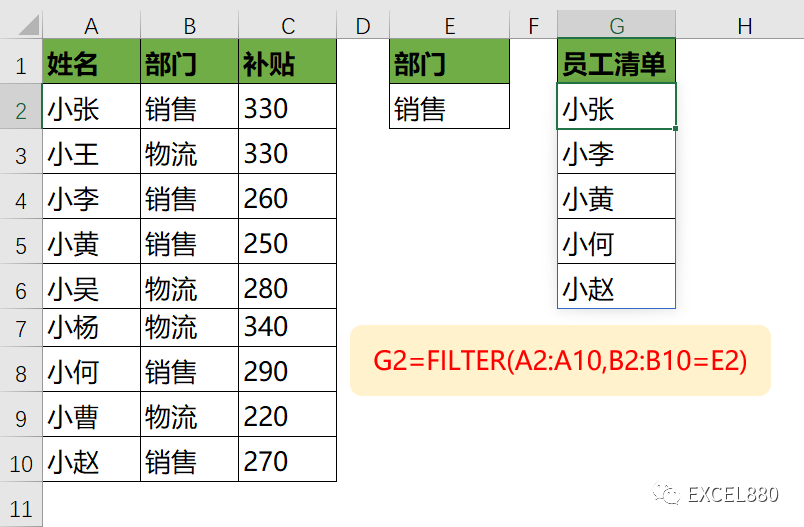=FILTER(A2:A10,(B2:B10=E2)*(C2:C10>300))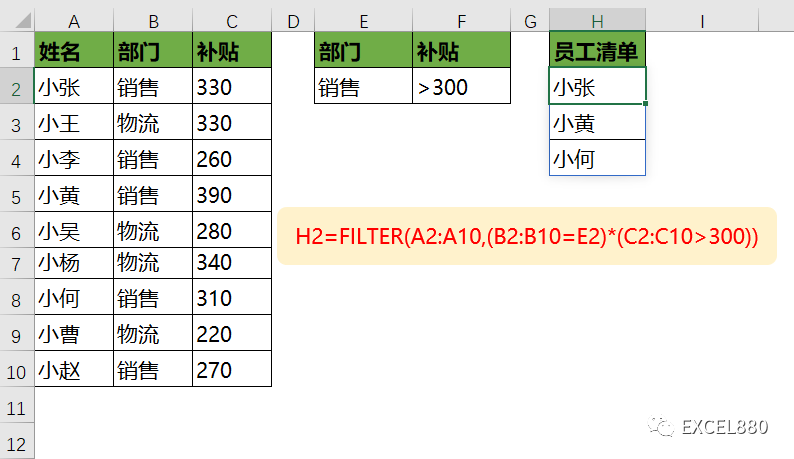=FILTER(A2:A10,ISNUMBER(FIND(F2,C2:C10)))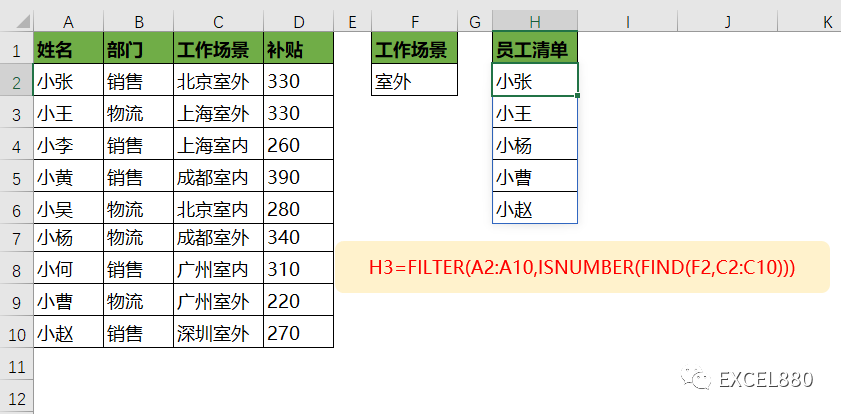=UNIQUE(FILTER(B2:B10,C2:C10=F2))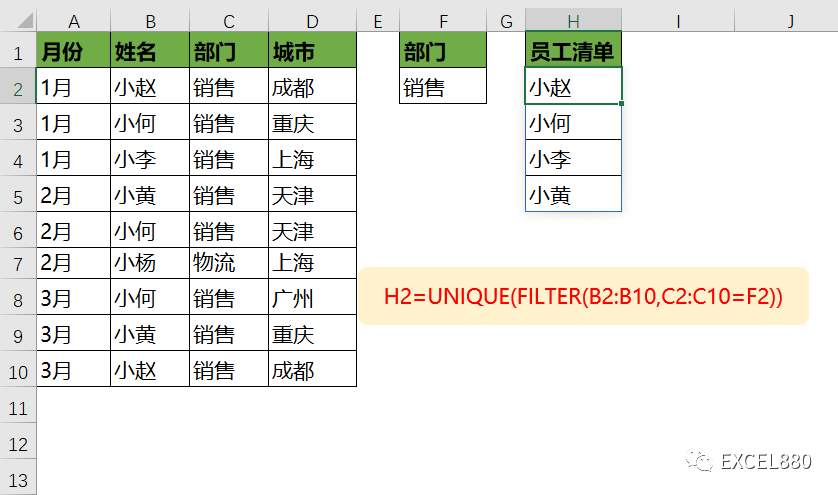=SORT(FILTER(A2:C10,B2:B10="销售"),3)